# AP Chemistry : Le Chatelier's Principle

## Example Questions

2 Next →

### Example Question #11 : Le Chatelier's Principle

Which of the following stresses to a system at equilibrium cause an increase in the production of CH3OH ? CO(g)+ 2H2(g) → CH3OH(g)
(a) H2 is added. (b) The volume is increased. (c) Argon is added. (d) Removing CO.

(a), (c), (d)

(a)

(a), (d)

(a), (b), (c), (d)

(b)

(a)

Explanation:

LeChatelier’s principle states that if a stress is applied to a rxn mixture at equilibrium,
reaction occurs in the direction that relieves the stress. Therefore, adding H2 will produce
more methanol. By increasing the volume and decreasing the pressure, there will be a net
reaction in the direction that increases the number of moles of gas. So since there are 3
moles of gas on the products side and only 1 mole of gas on the reactants side, if the volume  is increased less methanol will be produced. Since Ar is an inert, it will have no eﬀect on the amount of methanol produced. Removing CO will cause a shift in the reaction from right to left causing less methanol to be produced.

### Example Question #12 : Le Chatelier's Principle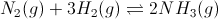Figure 1: Ammonia gas formation and equilibrium

Experimental data shows that the reaction shifts to the left at very cold temperatures. Using this information, what type of reaction is shown in Figure 1?

Exothermic

Exergonic

Boltzmann-like

Endothermic

Endergonic

Endothermic

Explanation:

This is an application of Le Chatlier's Principle. When you take away heat from the reaction, the reaction shifts toward the left in order to compensate from the heat loss. The reaction may then be rewritten to include energy as a reactant.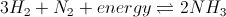Since the energy is on the reactant side, the reaction is endothermic.

### Example Question #13 : Le Chatelier's Principle

Which of the following will not shift the equilibrium of a reaction?

Increasing the temperature

Taking away products

Explanation:

A catalyst does not change the equilibrium of a reaction. Catalysts will affect reaction rate by lowering activation energy, but will ultimately have no effect on the amount of reactants and products present when equilibrium is reached.

Adding or removing reactants or products will result in a shift in equilibrium according to Le Chatelier's principle. Similarly, changing the temperature of a reaction will affect equilibrium in different ways depending on the enthalpy of reaction; increasing the temperature of an exothermic reaction will increase the reactant concentration, while increasing the temperature of an endothermic reaction will increase the products.

### Example Question #14 : Le Chatelier's Principle

Consider the following balanced chemical equation: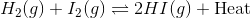What will be the effect on the concentration of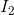if the overall pressure of the system increases, but the volume remains constant? Why?

There will be no effect onbecause the number of molecules of gas is the same on both sides

The concentration ofwill increase because that will counteract the increased pressure

The concentration ofwill decrease because that will counteract the increased pressure

The concentration ofwill increase because of added heat

The concentration ofwill decrease because of added heat

The concentration ofwill increase because of added heat

Explanation:

To answer this question we need to combine our knowledge of a few different subjects. Under normal cicrumstances, when determining the effects of a system pressure change, we compare the number of moles of gas on either side of the equilibrium. In this case, there are 2 moles of gas on either side, which means that neither side is favored in terms of pressure changes.However, we consider that in this case, the pressure is increasing while the volume remains constant. Since the total moles of gas cannot be changed in a closed system, we have to conclude that increased pressure results in increased temperature. If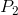is greater than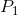, then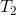must also be greater than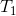.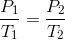Since this reaction is exothermic, increasing the temperature (i.e. adding heat) causes the reaction to shift to the left; therefore, the concentration ofincreases.

### Example Question #15 : Le Chatelier's Principle

Consider the following reaction:What will happen to the equilibrium constant if the concentration of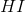is increased? Why?

Nothing, because the equilibrium constant is not affected by concentration changes

The equilibrium constant will increase because the concentration ofhas increased

The equilibrium constant will decrease because the reaction will shift towards the reactants

The equilibrium constant will go through an unclear change because it is temperature dependent

The equilibrium constant will go through an unclear change because it is temperature dependent

Explanation:

By Le Chatelier's principle, when a concentration on the right side increases, the reaction shifts towards the left side in order to balance out the disturbance to equilibrium. In doing so, heat will be consumed along with, which will lower the temperature of the system. Since the equilibrium constant is temperature dependent its value will change, but without experimental observation it is not entirely clear how it will change.

It is true that concentration changes normally do not affect the equilibrium constant, but because in this case temperature is affected by concentration changes, that rule does not hold.

### Example Question #16 : Le Chatelier's Principle

Anaerobic respiration is a very important process for humans; both in the sense of survival (in humans: lactic acid fermentation), and in the sense of recreation (the utilization of yeast to produce ethanol from glucose under anaerobic conditions). The following is the balanced chemical equation for the production of ethanol from glucose as is employed in the production of wine and beer: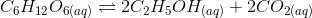This reaction is exothermic, and is therefore used by yeast to provide energy.

Assume the above reaction starts at equilibrium. Which of the following changes to the system will cause the reaction quotient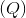to become less than the equilibrium constant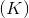?

Removal of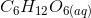Addition of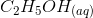Increasing the temperature of the system, thereby providing activation energy

Addition ofAddition of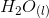thereby decreasing the concentration of products

Addition ofExplanation:

### General formula for the reaction quotient: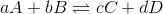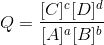Since the reaction starts at equilibrium the initial value of the reaction quotient is equal to the equilibrium constant.

When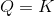, the reaction is at equilibrium.

When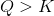, the reaction favors the reactant(s).

When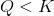, the reaction favors the product(s).

For this problem: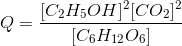Since the reaction started at equilibrium at this problem, initially. Therefore in order to make the(and therefore favor the production of products) we must alter the above equation for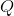to have a smaller value. There are many ways to do this. We can reduce the concentration or either or both of the products, and/or we can increase the concentration of reactant.

Removal ofdecreases the concentration of reactant and would causeAddition ofincreases the concentration of product and would causeIncreasing the temperature of the system, thereby providing activation energy is incorrect because this is an exothermic reaction and therefore energy (in this case in the form of heat) is considered a product and would cause. Also it is worth noting that changing the activation energy of a reaction only changes the rate of the reaction, not the direction in which it proceeds.

Addition ofthereby decreasing the concentration of products would not change the value of, because addingwill also decrease the concentration or the reactants by the same factor.

Addition ofis the only answer which will alter the value ofto be less than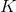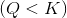, because addition ofwill increase the concentration of reactants. This increases the value of the denominator in the following equation :Since dividing by a larger number results in a lower value for, the addition ofresults in(when the reaction starts at equilibrium, if the reaction didn't start at equilibrium increasing the concentration of glucose will still lower the value of, but we would need more information to know if it decreased it enough to be less than.

### Example Question #17 : Le Chatelier's Principle

Consider the following reaction: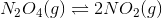Which of the following changes would be expected to drive the reaction to the left?

Reducing the amount of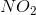in the reaction vessel

An increase in pressure

Addition of water to the reaction mixture

A decrease in pressure

An increase in pressure

Explanation:

For this question, we're given a reversible reaction and are asked to identify conditions which would drive the reaction towards the left. In order to answer this, we'll need to understand the fundamentals of Le Chatelier's principle. The basic idea of this principle is that whenever stress is added to a system that is in equilibrium, that system will establish a new equilibrium in order to handle the added stress.

In this question, we can see that both reactant and product are gases. However, they differ in amount. For every one mole of reactant, two moles of product are made. This is an important distinction that will allow us to get our answer. Whenever a system in equilibrium is composed of gases, increased pressure will favor the gas that can exist in a smaller amount. Conversely, decreased pressure will favor the gas that can exist in a greater amount. The relevance that this has to this question is that increased pressure will actually push the reaction to the left, since it is the reactant that can exist in fewer moles as a gas. A decrease in pressure would be expected to have the opposite effect, as it would increase the amount of product and thus would drive the reaction to the right. Reducing the amount of product in the reaction vessel would also be expected to have the opposite effect, as the system would respond by producing more product in order to help offset the loss. And finally, there is no indication of whether the addition of water would have any kind of effect on the reaction equilibrium in this question, thus there is no way to tell.

### Example Question #18 : Le Chatelier's Principle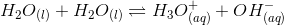Self-ionization of water is endothermic. What is the value of the sum pH + pOH at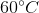?

Equal to 14

Less than 14

Greater than 14

Less than 14

Explanation:

Recall that ion-product constant of water,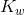, is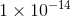at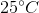and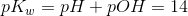.

An endothermic reaction signifies that heat is at the reactant side. By the LeChatelier's principle, increased heat toshifts the equilibrium to the right side, favoring the increase of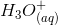and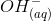. This means that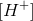and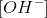both increase, decreasing pH and pOH to less than 7, each. As a result, pH + pOH is less than 14.

### Example Question #19 : Le Chatelier's Principle

Which of the following does not affect the equilbrium of a reaction?